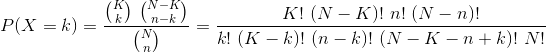primefactr

R package that uses Prime Factorization for computations.

This small R package was initially developed to compute hypergeometric probabilities which are used in Fisher’s exact test, for instance.

Installation and Attachment

## Installation
install.packages("primefactr")

## Attachment
library("primefactr")

Features

Main feature

To computeyou can use ComputeDivFact(c(K, (N-K), n, (N-n)), c(k, (K-k), (n-k), (N-K-n+k), N)). This uses a Prime Factorization to simplify computations.

Play with primes

You can also test if a number is a prime and get all prime numbers up to a certain number.